# Fluid Mechanics

Stream line flow: - Flow of a liquid fluid is said to be streamlined if the velocity of a molecule, at any point, coincides with that of the preceding one.

Laminar flow: - It is a special case of streamline flow in which velocities of all the molecules on one streamline is same throughout its motion.

Turbulent flow: - Whenever the velocity of a fluid is very high or it rushes past an obstacle so that there is a sudden change in its direction of motion, the motion of fluid becomes irregular, forming eddies or whirlpools. This type of motion of fluid is called turbulent flow.

Rate of flow (Equation of continuity): -

AV = Constant, A – Area, V – velocity

A1V1=A2V2

Equation of continuity can be considered to be a statement of conservation of mass.

So, V 1/A

Velocity of flow of liquid varies inversely as the area of cross-section of the opening from where the liquid comes out.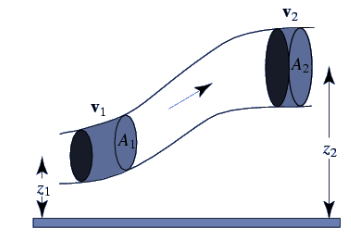(a) Kinetic Energy: - It is the energy possessed by a liquid by virtue of its velocity.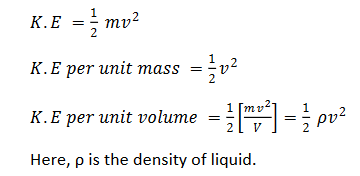(b) Potential Energy: - It is the energy possessed by a liquid by virtue of its position.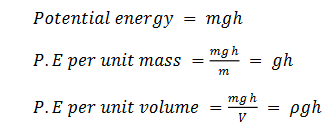(c) Pressure Energy: - It is the energy possessed by a liquid by virtue of its pressure.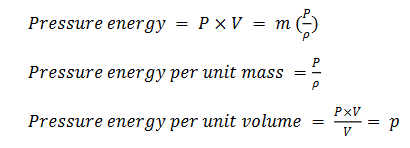Total energy of a liquid is the sum total of kinetic energy, potential energy and pressure energy.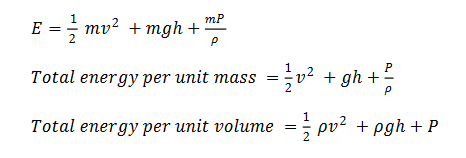Bernoulli’s Equation: -

It states that the total energy of a small amount of an incompressible non-viscous liquid flowing without friction from one point to another, in a streamlined flow, remains constant throughout the displacement.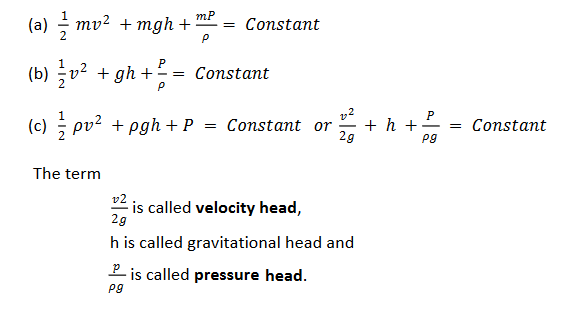Therefore, Bernoulli’s theorem states that in case of an incompressible, non-viscous fluid, flowing from one point to another in a streamlined flow, the sum total of velocity head, gravitational head and the pressure head is a constant quantity.

Torricelli’s theorem (velocity of efflux)

It states that the velocity of efflux of a liquid (V), from an orifice, is equal to the velocity acquired by a body, falling freely (v), from the surface of liquid to the orifice.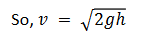• Viscosity: - Viscosity is the property of fluids by virtue of which they tend to destroy any relative motion between their layers.

• Velocity Gradient: - Velocity gradient is defined as the rate of change of velocity with respect to distance.

Newton’s law of Viscosity

In accordance to Newton’s law of viscosity, the viscous drag force depends upon the nature of fluid along with following factors: -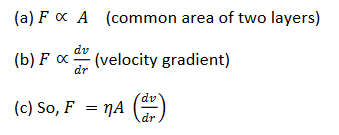Unit of η: -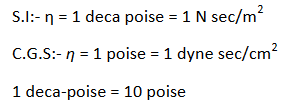Fluidity: - Reciprocal of coefficient of viscosity of a fluid is called its fluidity.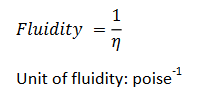Kinematic viscosity: - Kinematic viscosity of a fluid is defined as the ration between its coefficient of viscosity to the density of fluid.

Kinetic viscosity of a fluid having its dynamic viscosity one poise and density one g cm-3 is said to be 1 stoke.

Critical velocity (Reynold’s Number)

Critical velocity (vc) is the maximum velocity of the flow of liquid flowing in a streamlined flow.

𝑣𝑐`= 𝑁𝑅𝜂/𝜌𝐷

Here η is the coefficient of viscosity of liquid, ρ is the density of liquid and D is the diameter of the tube.

Reynold’s Number, 𝑁𝑅 = 𝜌𝑣𝑐𝐷/𝜂

Stokes Law: - In accordance to Stokes’ law, force of viscosity F depend upon, force of viscosity,

𝐹=6𝜋𝜂𝑟𝑣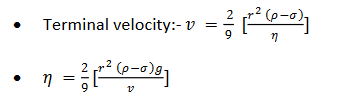(a) Effect of temperature on η: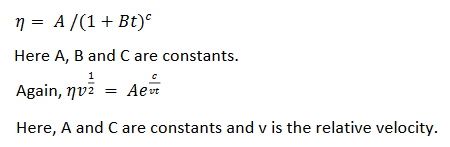(b) Effect of pressure on η: Co-efficient of viscosity of liquids increases due to an increase in pressure but there is no relation, so far, to explain the effect.

Change in viscosity of gases: -

(a) Effect of temperature: - Co-efficient of viscosity of a gas at a given temperature is given by,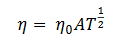Here T is the absolute temperature of gas.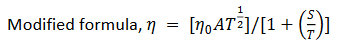(b) Effect of pressure: - At low pressure, co-efficient of viscosity of a gas varies directly with pressure.

1. Rate of flow of liquid through a liquid through a capillary tube of radius r and length l,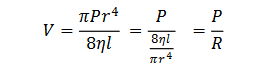Pressure exerted by a column of liquid of height h: - 𝑃 = h𝜌𝑔

Here, ρ is the density of liquid.

2. Pressure at a point within the liquid: - 𝑃 = 𝑃0 + h𝜌𝑔

Here, P0 is the atmospheric pressure and the depth of point with respect to free surface of liquid.

Post By : Ravindra Yadav 03 Nov, 2019 3182 views Physics